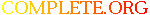Complete.Org: Mailing Lists: Archives: freeciv-ai: November 2002: [freeciv-ai] Re: Measure the AI ability to harvest resources# [freeciv-ai] Re: Measure the AI ability to harvest resources

[Top] [All Lists]

 To: freeciv-ai@xxxxxxxxxxx Subject: [freeciv-ai] Re: Measure the AI ability to harvest resources From: Raimar Falke Date: Mon, 25 Nov 2002 09:59:26 +0100

```On Mon, Nov 25, 2002 at 12:48:21AM +0100, Raimar Falke wrote:
> > 2: 29:            Wang Gon:(f s t)=(  71  147  150) (l t s)=(   0   42
> > 108) s=  5648 uf=4000
> > 2: 29:      Guntis Ulmanis:(f s t)=( 123  160  162) (l t s)=(   0   11
> > 151) s=  7001 uf=4000
> > 2: 29:   Richard Lionheart:(f s t)=( 108  195   92) (l t s)=(   0   30
> > 62) s=  6471 uf=4000
> > 2: 29:             Genghis:(f s t)=( 145  173  139) (l t s)=(   0   19
> > 120) s=  7364 uf=4000
>
> 2: 29:Wang Gon
> 2:               Settlers: 1 * 40 = 40
> 2:               Warriors: 1 * 10 = 10
> 2:               Barracks: 2 * 40 = 80
> 2:   total = 130 shields
> 2: 29:Guntis Ulmanis
> 2:               Settlers: 2 * 40 = 80
> 2:               Barracks: 1 * 40 = 40
> 2:   total = 120 shields
> 2: 29:Richard Lionheart
> 2:               Settlers: 3 * 40 = 120
> 2:               Warriors: 1 * 10 = 10
> 2:               Barracks: 1 * 40 = 40
> 2:   total = 170 shields
> 2: 29:Genghis
> 2:               Settlers: 3 * 40 = 120
> 2:               Barracks: 1 * 40 = 40
> 2:   total = 160 shields

Same game after 100 turns (1AD):

2: 99:            Wang Gon:(f s t)=( 440  856  795) (l t s)=(   0  272  523) s=
32452 uf=4000
2: 99:      Guntis Ulmanis:(f s t)=(1119 2917 1369) (l t s)=(   0  700  669) s=
87278 uf=4000
2: 99:   Richard Lionheart:(f s t)=(1271 2974  647) (l t s)=(   0  444  224) s=
82471 uf=4000
2: 99:             Genghis:(f s t)=(1273 2532  984) (l t s)=(   0  514  430) s=
79039 uf=4000
2: 99:Wang Gon
2:               Settlers: 11 * 40 = 440
2:               Warriors: 13 * 10 = 130
2:               Barracks: 4 * 40 = 160
2:   total = 730 shields
2: 99:Guntis Ulmanis
2:               Settlers: 19 * 40 = 760
2:               Warriors: 1 * 10 = 10
2:               Diplomat: 22 * 30 = 660
2:               Barracks: 19 * 40 = 760
2:             Courthouse: 5 * 80 = 400
2:   total = 2590 shields
2: 99:Richard Lionheart
2:               Settlers: 43 * 40 = 1720
2:               Warriors: 1 * 10 = 10
2:               Barracks: 20 * 40 = 800
2:   total = 2530 shields
2: 99:Genghis
2:               Settlers: 36 * 40 = 1440
2:               Warriors: 17 * 10 = 170
2:               Barracks: 17 * 40 = 680
2:   total = 2290 shields

No capitalization.

Raimar

--
email: rf13@xxxxxxxxxxxxxxxxx
"I do feel kind of sorry for Microsoft. Their attornies and marketing
force must have tons of ulcers trying to figure out how to beat (not
just co-exist with) a product that has no clearly defined (read
suable) human owner, and that changes on an hourly basis like the
sea changes the layout of the sand on a beach. Severely tough to
fight something like that."
-- David D.W. Downey at linux-kernel

```